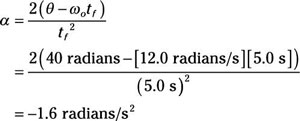##### Physics I Workbook For Dummies with Online Practice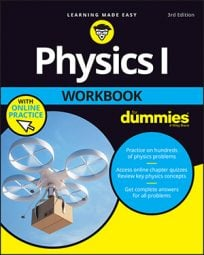You can connect angular velocity, angular acceleration, and time to angular displacement. This is very similar to the way that you connect linear velocity, linear acceleration, and time to linear displacement. Recall that you can connect displacement to the original velocity and linear acceleration like this: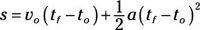And you can make the substitution from linear to angular motion by putting in the appropriate symbols: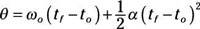Using this equation, you can connect angular velocity, angular acceleration, and time to the angle.

## Sample question

1. A marble is rolling around a circular track at 6.0 radians/s and then accelerates at 1.0 radians/s2. How many radians has it gone through in 1minute?

1. Use this equation:2. Plug in the numbers: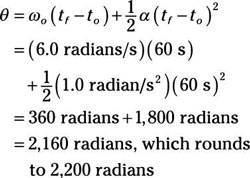## Practice questions

1. Your model globe is spinning at 1.0 radians/s when you give it a push. If you accelerate it at 5.0 radians/s2, how many radians has it turned through in 5.0 s?

2. Your toy plane on a wire is traveling around in a circle at 8.0 radians/s. If you accelerate it at 1.0 radians/s2 for 20.0 s, how many radians has it gone through during that time?

3. You're whipping a ball on a string around in a circle. If it's going 7.0 radians/s and at the end of 6.0 s has gone through 60.0 radians, what was its angular acceleration?

4. A roulette wheel is slowing down, starting from 12.0 radians/s and going through 40.0 radians in 5.0 s. What was its angular acceleration?

Following are answers to the practice questions:

1. Use this equation:2. Plug in the numbers: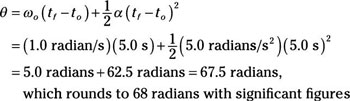1. Use this equation:2. Plug in the numbers: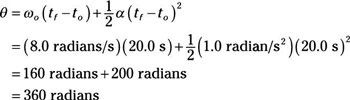1. Use this equation:2. Solve for alpha, given that to = 0: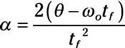3. Plug in the numbers: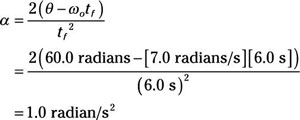1. Use this equation:2. Solve for alpha, given that to = 0:3. Plug in the numbers: